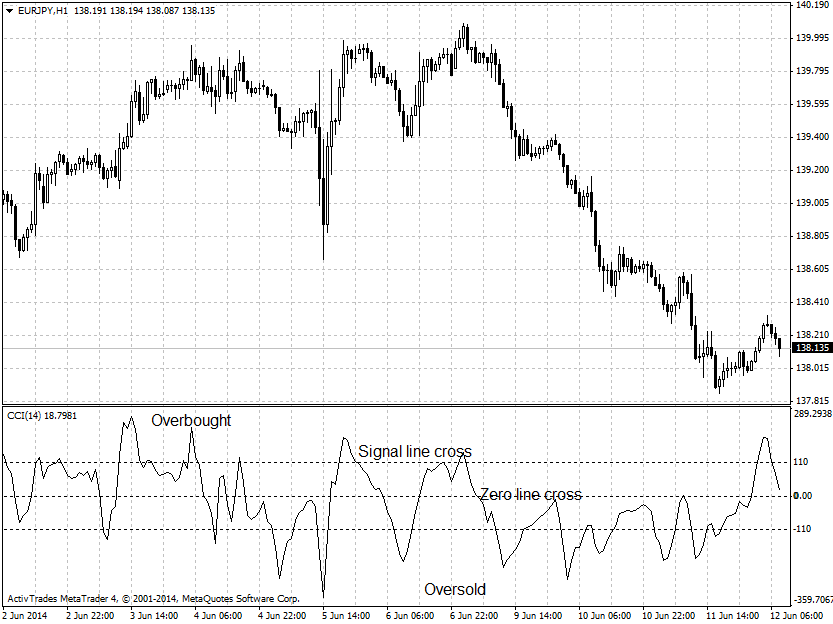# forex software

Create and Test Forex Strategies

forex software

trading:cci

# Commodity Channel Index - CCI

The commodity channel index (CCI) is an oscillator developed by Donald Lambert and is used for analysis only of the commodities markets, but also equities and currencies. This indicator measures the variation of the price from a moving average. The CCI is calculated as the difference between the typical price (average of the high, low and close of the period) and its simple moving average, divided by the mean deviation of the typical price. The index is usually scaled by an inverse factor of 0.015. Lambert set the factor at 0.015 to ensure that approximately 70 to 80 percent of CCI values would fall between −100 and +100. Signal lines are usually placed at these two levels, but if you want to filter some of the set ups you can use 110 and -110 levels instead.

CCI is calculated by the following formula:

```          Typical Price - SMA (n)
CCI = ------------------------------
0.015 * Deviation```

CCI is generating the following signals:

• Values above the Zero line - trend is up
• Values below Zero line - trend is down
• Extreme levels above 100/below -100 indicate overbought/oversold market conditions
• Cross below 100 line gives sell signal
• Cross above -100 line gives buy signal
• Hook at Zero line signals the beginning of a new leg of the trend
• Divergence of the price and indicator charts indicates possible trend reversal